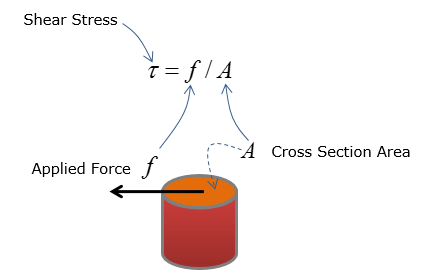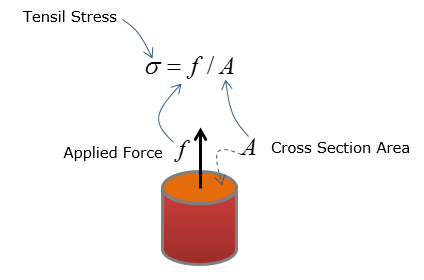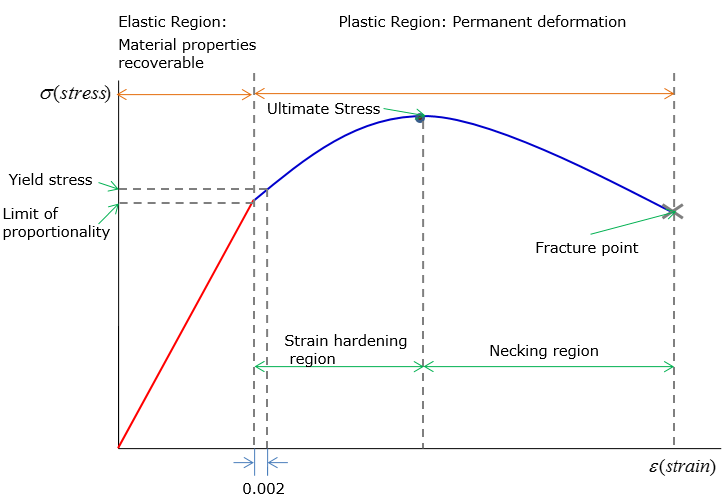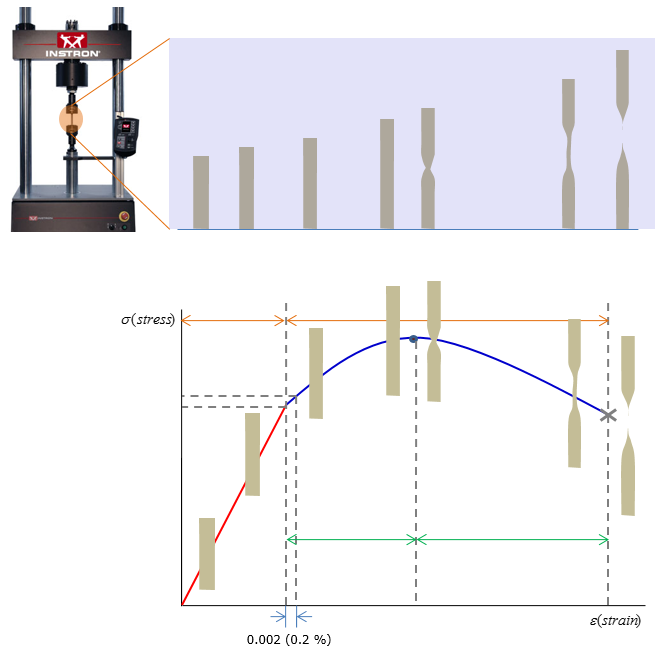Mechanical Engineering - Solid Mechanics                                 Home : www.sharetechnote.com Stress and Strain     < Stress >   Stress is the intensity of force over an area and it can be categorized as shear stress and tensile stress   Shear stress: Applied force is parallel to the cross-section of the object Equation:Tensile stress: Applied force is perpendicular to the cross-section of the object Equation:< Strain >   Strain is quantified as change in length over initial length Strain can be induced by both applied force and change in temperature     < Stress and strain relationship and application >   Stress-strain curve is used to analyze the deformation characteristics of a material under applied force – straining of the object induce stress: refer to the next slide for the curve Stress in a support system is calculated based on strain characteristics of the rigid body